Damage assessment of shear wall components for RC frameĘCshear wall buildings using story curvature as engineering demand parameter Chen Xiong a, Xinzheng Lu b,*, Xuchuan Lin c,d a Guangdong Provincial Key Laboratory of Durability for Marine Civil Engineering, Shenzhen University, Shenzhen, China. b Key Laboratory of Civil Engineering Safety and Durability of China Education Ministry, Department of Civil Engineering, Tsinghua University, Beijing, China. c Institute of Engineering Mechanics, China Earthquake Administration, Harbin, China. d Key Laboratory of Earthquake Engineering and Engineering Vibration, China Earthquake Administration, Harbin, China. Engineering Structures, 2019, 189: 77-88. DOI: 10.1016/j.engstruct.2019.03.068. Download Full Text PDF Abstract: Reinforced concrete (RC) frameĘCshear wall structures are extensively used in urban areas. As a major part of the lateral load resisting system, the shear wall is a key component for the seismic performance assessment of such structures. In this study, a method for the seismic damage assessment of shear wall components is proposed by using the story curvature as the engineering demand parameter (EDP). The method involves (1) a shear wall story curvature calculation method and (2) a shear wall damage limit determination method that uses the story curvatures as the EDP. The story curvature adopted in this study denotes the maximum shear wall curvature within each story level. Based on the piecewise linear assumption of the curvature distribution, the story curvature calculation method requires story drifts and shear wall key design parameters as inputs. Meanwhile, based on the plane cross-sectional assumption and sectional analysis, the damage limits can be determined by considering the influences of the shear wall key design parameters, such as the axial load ratio, shear wall component length, and material information, thereby yielding more accurate estimation. Finally, the proposed method is validated by comparing it with the numerical results of several wall panels and an RC frameĘCshear wall structure. It is further validated by comparing it with the experiment results obtained from six shear wall tests and a full-scale shaking table test of a seven-story shear wall structure. Key words: RC shear wall; Story curvature; Damage assessment; Sectional analysis.
 1. Introduction Reinforced concrete (RC) frameĘCshear wall structures are extensively used in urban areas for office and residential buildings. Sheltering numerous city functionalities and a large number of people, RC frameĘCshear wall structures in urban areas play an important role. RC frameĘCshear wall structures typically exhibit a good collapse prevention performance , but the seismic damages of such structures can still cause severe economic losses. For example, in the 2011 Christchurch Earthquake, a number of RC frameĘCshear wall buildings were severely damaged. Because the damages of shear wall components are very difficult to repair, numerous buildings were demolished, resulting in considerable direct and indirect losses to the area [2,3]. The performance-based  and resilience-based  seismic designs of RC frameĘCshear wall structures are effective measures for addressing the aforementioned problems, and both of the methods rely on an accurate seismic damage assessment of shear wall components. The seismic damage assessment method of shear wall components mainly involves two parts, namely, (1) engineering demand parameter (EDP) calculation and (2) damage limit determination. The details of these two parts are presented in the following. (1) EDP calculation The EDPs adopted for the damage assessment of shear wall components mainly have three categories, namely, material-, component-, and story-level EDPs. The material strain is a common material-level EDP for damage assessment of shear wall panels. The strain of different materials can be obtained from the numerical simulations of refined finite element (FE) models or experimental measurements. Using the material-level EDP is a straightforward approach, and the EDP can clearly reflect the damage of the corresponding material. For example, Yang et al.  conducted a damage assessment for a 42-story high-rise building, in which the compressive strain was adopted as an EDP to assess the damage state of shear wall boundary elements. However, owing to the different strains in various parts of the shear wall panel, determining the damage states of a specific story based on the strains is not easy. ASCE 41  recommended two component-level EDPs for the damage assessment of shear walls. The first one is the demand capacity ratio (DCR) and the second one is the plastic hinge rotation. The DCR is defined as the ratio of the component demand to the component strength. Yang et al.  adopted the maximum shear DCR as the EDP to assess the shear wall of a 42-story building. Birely  also utilized the DCR values of shear, moment, and axial demand to assess the damage states of four high-rise buildings. However, the DCR is often calculated on the basis of the action demands from a linear static procedure (LSP) or linear dynamic procedure (LDP), which cannot capture the nonlinear behavior of shear walls. The plastic hinge rotation is also recommended in ASCE 41  as the component-level EDP. ASCE 41  specified the computational method and the damage limits for the plastic hinge rotation at the bottom of the shear wall, but the damages at the upper part of the shear wall panel cannot be considered. Note that owing to the significant contribution of high-order vibration modes of tall buildings, damages located at the upper parts of tall buildings were frequently observed in numerical simulations . Story-level EDPs are simple and are extensively used for the seismic damage assessment of individual and urban buildings [8-14]. The data required to compute the story-level EDP can be easily obtained from numerical simulations or structural health monitoring. One of the most frequently used story-level EDPs is the inter-story drift ratio (IDR). The IDR can be used to determine the story-level damages of multi-story buildings that exhibit shear deformation modes. However, it is inappropriate for the damage assessments of shear wall components of high-rise buildings because the responses of these buildings follow a flexuralĘCshear deformation mode, and a large proportion of the IDR at the upper story is contributed by the rigid-body rotation . To address this problem, a new story-level EDP, called inter-story pure translation ratio (ISPT), is presented by Ji et al. , as shown in Eq. 1.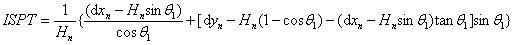(1) where Hn is the height of the nth story; dxn and dyn are the horizontal/vertical inter-story displacements, respectively; and q1 is the bottom rotation of the nth story. The ISPT can effectively remove the rigid-body rotation and is reliable for the damage assessment of shear wall components. It has also been adopted by Alwaeli et al.  to assess the seismic damage of RC high-rise wall buildings. In the seismic damage assessment method of FEMA P58 , the effective drift ratio is adopted as the EDP of the slender concrete shear walls. The effective drift ratio is defined as the ratio of the lateral drift at the effective height to the effective height. The effect height of a shear wall can be calculated as the ratio of the base moment to the base shear. The fragility data of the slender concrete shear walls are also provided in the FEMA P58 fragility database, which can be used to assess the damages of the concrete shear walls reliably. (2) Damage limit determination The drift ratio (DR) results of shear wall experiments, defined as shown in Fig. 1 and Eq. 2, are typically used as the basis to determine the damage limits of shear wall components . Notably, directly using the DR limits of shear wall specimens as the IDR criteria for buildings is sometimes inappropriate. The reason is that the aspect ratio of a slender shear wall specimen often differs from the story height to shear wall length ratio, as shown in Fig. 1. Therefore, the DR of a shear wall specimen is not equivalent to the IDR of a building, as expressed by Eqs. 2 and 3, respectively.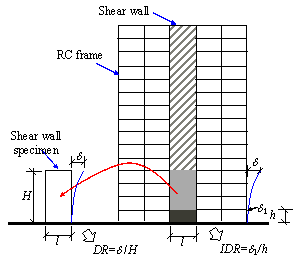Fig. 1. Comparison of DR and IDR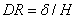(2)(3) where d is the top displacement of the shear wall specimen, d1 is the inter-story displacement, H is the height of the shear wall specimen, and h is the story height. Moreover, the damage limits of shear walls are influenced by axial load ratio, material properties, length of the shear wall, etc. According to the statistical results of shear wall experiments, the maximum DR limit of a specific damage state for different shear wall specimens can be up to 10 times higher than the minimum one . Therefore, the statistical results of damage limits for specimens with various design parameters can be extremely dispersed. Consequently, assessing the damage states of a shear wall component by using the dispersed damage limits can lead to unreliable results [17,18]. Although shear failure or flexure-shear failure can be observed in shear walls in certain cases , most shear wall components are expected to fail in flexure, because the shear strength of a shear wall is guaranteed according to the capacity design principle of shear wall components . Therefore, the method proposed in this study mainly focuses on assessing the flexural damage of shear walls. Note that the flexural failure is strongly related to the component curvature . The curvature distribution in shear wall components is adopted to estimate the damage state at different story levels of a shear wall component. Specifically, the maximum curvature within each story level is adopted as the story curvature EDP in this study to assess the story-level seismic damage of the shear wall components, and the proposed method can be very useful when it is applied in simplified nonlinear methodologies for building seismic performance assessment . The method proposed in this study is characterized by the following features: (1) the proposed method can consider the damage in the middle region of a shear wall, caused by higher-order building vibration. However, certain conventional methods, for example, the method that adopts the effective drift ratio as the EDP, can only consider the damage at the bottom of shear walls, induced by first-order building vibration. (2) The proposed method can estimate the shear wall damage at all story levels, which facilitates the future repair time and repair cost simulation . (3) The damage limit determination method presented in this study can make full use of the key design parameters of a shear wall, thereby yielding more reliable damage limits. In particular, a story curvature calculation method is proposed in Section 2. Based on the piecewise linear assumption of the curvature distribution, the story curvature can be obtained with story drifts and several shear wall key design parameters as inputs, which is best suited to cases in which shear wall rotation distribution data are difficult to obtain (for example, structural health monitoring). A damage limit determination method is presented in Section 3. Based on the plane cross-sectional assumption and sectional analysis, the method can consider the influences of the axial load ratio, shear wall length, and material information, thereby yielding more accurate damage limits. In Section 4, the proposed methods are validated by means of comparison with the numerical results obtained from several shear wall panels and from a shear wall structure. Furthermore, the effectiveness of the method is demonstrated by means of comparison with the damage assessment method of FEMA P58 in Section 4.2. The methods are further validated by comparison with the experimental results obtained from six shear wall specimens as well as the shaking table test results of a seven-story shear wall building. The outcomes of this work are expected to provide a useful reference for performance-based  and resilience-based  seismic design in the cases of RC frameĘCshear wall structures.

2. Story curvature calculation

This study aims to assess the story-level seismic damage of shear wall components. Hence, the story curvature referred to in this study indicates the maximum curvature of each story of shear wall components.

The flexural shear model presented in Fig. 2a is widely used for the design and simulation of RC high-rise buildings [23-26]. In this model, the shear wall components are simulated with EulerĘCBernoulli beams and the frame components are modeled with shear springs. The shear wall and frame components are connected with rigid links, and the moment transfer between the shear wall and frame components is ignored . In the study of Kuang and Huang , this model is proved to be reliable in simulating the nonlinear responses of regular wallĘCframe structures. The model of the shear wall component is illustrated in Fig. 2b. Note that although the actual structure of a tall building is much more complicated than the simplified one in Fig. 2a, many previous works have proofed that such model can accurately represent the dynamic responses of various tall buildings [23-26].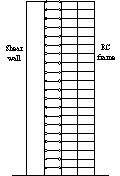(a) A simplified model of RC frameĘCshear wall buildings (b) A simplified model of shear walls components

Fig. 2. A simplified model for RC frameĘCshear wall structures and shear wall components

2.1 Story rotation calculation

The story rotation calculation method is proposed for the cases in which the rotation data of shear wall components are difficult to obtain. For the cases in which story rotation data are easy to acquire, this section can be skipped and attention is redirected to Section 2.2 for the story curvature calculation.

As shown in Fig. 2b, each story of the simplified shear wall component model is consisted of one EulerĘCBernoulli beam and one mass point. The mass point has one lateral translational degree of freedom (DOF) and one rotational DOF. The external force acting on each mass point is the lateral force Fi and the moment Mi. The dynamic equilibrium equation of the simplified model in Fig. 2b can be presented in Eq. 4.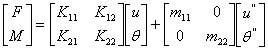(4)

where F and M are the external force/moment vectors acting on each mass point, u and q are the lateral and rotation displacement vectors, u'' and q '' are the lateral and angular acceleration vectors, m11 and m22 are the partitioned mass matrices, and K11, K12, K21, and K22 are the partitioned stiffness matrices of the global stiffness matrix K. Note that when the maximum story curvature is calculated, the story displacement reaches the maximum value and the velocity of each story approaches zero. Therefore, the viscous damping term is ignored in Eq. 4.

The global stiffness matrix K is expressed in Eq. 5 for the case of a three-story shear wall building.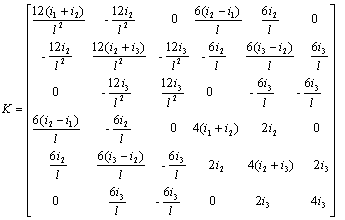(5)

where in=EIn/l, n is the story label, and l is the height of each story.

According to the assumption of the simplified shear wall model, the moment that is transferred between the shear wall and frame components can be ignored . Meanwhile, the moment contributed by the angular acceleration is also very minimal and can be ignored . Therefore, the static condensation technique can be applied on the rotational DOF, as indicated in Eq. 6.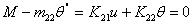(6)

By using Eq. 6, the relationship between the story rotations and story drifts can be expressed in accordance with Eq. 7.(7)

Therefore, given the story drifts, the story rotations can be computed using Eq. 7. To implement the story rotation calculation process, the partitioned matrices K22 and K21 should be obtained. Note that u and q in Eq. 7 denote the total displacement and the total rotation, respectively. Therefore, the EIn in Eq. 5 is the secant flexural stiffness of each story.

To obtain the partitioned matrices K22 and K21, the secant flexural stiffness EIn is assumed to be constant along the height of a single story. For example, in the elastic state (DS0) of a shear wall component, the cross-sectional dimension is identical in a story. Therefore, the above assumption is reasonable. However, when entering the inelastic phase, the plastic hinge often occurs in the wall panel and the secant flexural stiffness decreases. Considering that the length of the shear wall is typically larger than the story height, the length of the plastic hinge is often comparable to the story height . Therefore, the above assumption is also reasonable for the inelastic phase of a shear wall component.

On the basis of the above assumption, the elastic stiffness EI0n can be calculated according to the dimension of the wall panel. The inelastic secant stiffness EIn can be computed according to Eq. 8.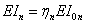(8)

where hn is the secant stiffness reduction factor.

To obtain the secant stiffness reduction factor hn, the nonlinear state of each story should be determined. In this part, three damage states are adopted, namely, none (DS0), cracking (DS1), and yielding (DS2). The damage limits between the three limit states are the cracking and yielding limits and the detailed discussion of these damage limits are presented in Section 3.

Based on the adopted three damage states, it is assumed that the shear wall remains elastic before cracking and the flexural secant stiffness is EI0n. After cracking, the secant stiffness gradually decreases and the flexural secant stiffness will be hEI0n, as shown in Fig. 3a. Hence, the secant stiffness reduction factor  h = 1 prior to the cracking curvature limit kcrack. Subsequently, the secant stiffness reduction factor h decreases rapidly and can be represented by a linear function  (Fig. 3b). When the yielding curvature limit kyield is reached, the yielding secant stiffness reduction factor hyield can be determined in accordance with ACIĘC318 . For example, for the RC shear wall components, hyield = 0.35. After the yield point, the strength can be assumed to be constant , as shown in Fig. 3a. Consequently, the secant stiffness reduction factor h becomes inversely proportional to the curvature (Fig. 3b). Based on the above discussion, the secant stiffness reduction factor h can be expressed as shown in Eq. 9.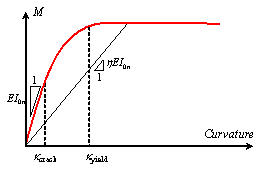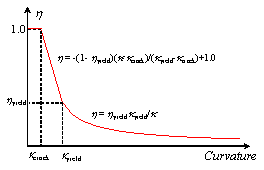(a) Illustration of h at different curvature conditions (b) Relationship between h and curvature

Fig. 3. Determination of the secant stiffness reduction factor h(9)

where k is the curvature of a shear wall section, kcrack is the cracking limit, kyield is the yielding limit, and hyield is the secant stiffness reduction factor at the yield point. The story curvature k can be computed according to story rotations, which will be covered in Section 2.2. The cracking and yielding curvature limits kcrack and kyield, respectively, will be discussed in Sections 3.1 and 3.2.

Based on the above, the secant flexural stiffness EIn of each story should be determined to calculate the story rotations. Note that the secant flexural stiffness EIn is related to the story curvatures, whereas the story curvatures can only be computed when the story rotations are given. Therefore, the above problem should be solved through an iterative process, as shown in Fig. 4.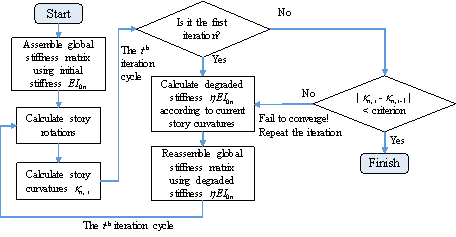Fig. 4. Iteration process of the story rotation calculation

2.2 Story curvature calculation

Given the story rotations, the mean story curvatures can be calculated according to Eq. 10.(10)

where kn, mean is the mean story curvature of the nth story and qn is the rotation at the top of the nth story, when n = 1, qn-1 = 0.

To obtain the curvature distribution, the secant flexural stiffness is assumed constant within a story, based on the discussion in Section 2.1. Because the shear force in a story is constant, the curvature within a story is linearly distributed, as illustrated in Fig. 5. Note that, in reality, the curvature distribution of a shear wall panel may be very complicated. Factors such as the occurrence of cracks, constraints of slabs and beams, and longitudinal reinforcement bond slip in shear walls may influence the curvature distribution in shear walls. It should be noted that this part mainly focuses on predicting the trend of the curvature distribution of shear wall panels and assessing the story-level damage of shear walls. The aforementioned factors that influence the curvature distribution in shear walls require further consideration in future studies. The effectiveness of the piecewise linear distribution adopted in this study is further demonstrated in Section 4.2.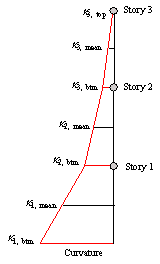Fig. 5.  Curvature distribution along the height of a shear wall component

As presented in Fig. 5, the mean story curvature kn,mean calculated using Eq. 10 is located at the mid-point of each story. Note that the maximum curvature of each story is often located at the top or bottom of this story. The curvature at the bottom of each story can be calculated using Eq. 11.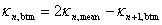(11)

where kn, btm is the story curvature at the bottom of the nth story. For the top story, kn,top = kn+1,btm = 0.

After the story curvature at the bottom of each story is obtained, the maximum curvature of the story can be computed using Eq. 12.(12)

Based on the aforementioned processes, the maximum story curvatures can be calculated. Combined with the damage limits obtained from the following section, the damage states of a shear wall panel on different stories can be easily estimated.

3. Determination of damage limits

The extent of damage of a building or a structural component can be described on the basis of different damage states. FEMA 273 and ASCE 41 adopted three building performance levels, namely, immediate occupancy (IO), life safety (LS), and collapse prevention (CP) [7,31]. HAZUS specified five damage states, namely, none, slight, moderate, extensive, and complete . Moreover, FEMA P58 recommended 24 different damage states for structural components . Considering the versatility and applicability of the proposed method, three damage limits with clear damage characteristics are adopted, namely, (1) the states when flexural cracking occurred, (2) when tensile or compressive reinforcement yielded, and (3) when concrete crushed or tensile reinforcement fractured. These damage states correspond to DS1b, (DS1d or DS1e), and (DS4a or DS4e), respectively, of FEMA P58 . The three damage limits divide the damages of a shear wall component into four states, that is, none (DS0), cracking (DS1), yielding (DS2), and failure (DS3). Considering that the main purpose of this work is the general method presentation, the story curvature limits of other damage states can also be determined using the following method.

3.1 Story curvature limit of the flexural crack point (DS1)

With the curvature development of the shear wall components, the edge of the shear wall cross section reaches the tensile strength of the concrete et. Based on the plane cross-sectional assumption, the strain distribution can be illustrated in Fig. 6, which illustrates the front view of the component with the length l. Lines AB and CD are the strain distributions of the section after and before the action of the lateral load, respectively. In Line CD, the section is subjected to the axial load, and the strain is distributed uniformly with the compressive strain ec0. With the increase of the lateral load, the strain distribution gradually transforms from Line CD to Line AB. After the tensile strain of Point A reaches the cracking strain et of concrete material, the section cracks. Therefore, the story curvature limit of the flexural crack point kcrack can be determined using Eqs. 13ĘC15.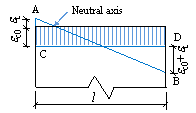Fig. 6. Strain distribution of the shear wall section at the flexural crack point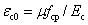(13)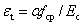(14)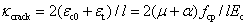(15)

where m is the axial load ratio, a is the ratio of the concrete tensile strength to the compressive strength, Ec is the Youngí»s modulus of the concrete, and fcp is the compressive strength of the concrete.

3.2 Story curvature limit of the yield point (DS2)

According to the plane cross-sectional assumption, the strain distribution of the shear wall section can be illustrated in Fig. 7a, where c is the depth of the compression zone, econ and eten are the compressive and tensile strains at the edge of the section, respectively. In addition, le is the effective length of the shear wall section that denotes the distance of longitudinal reinforcements at the tensile and compressive edges of the section.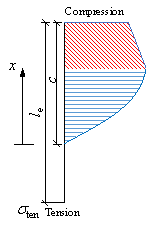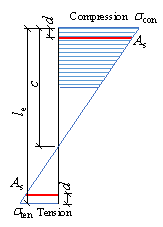(a) Strain distribution (b) Concrete stress distribution (c) Reinforcement stress distribution

Fig. 7. Strain and stress distributions of the shear wall section at the yield point

In this study, the yield point is assumed to be the moment when the maximum compressive strain econ or the maximum tensile strain eten reaches the yield strain ey of steel reinforcement. Therefore, the story curvature limit of the yield point kyield can be calculated using Eq. 16.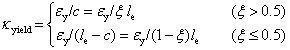(16)

where x = c/le is the relative depth of the compression zone.

Based on the above discussion, determining x is the key to calculate the story curvature limit of the yield point kyield. The relative depth of the compression zone x can be obtained by calculating the equilibrium equation of the section , as shown in Eq. 17.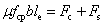(17)

where m is the axial load ratio, b is the thickness of the shear wall, and Fc and Fs are the forces contributed by the concrete and reinforcement parts, respectively, as shown in Figs. 7b and 7c.

The internal normal force Fc contributed by the concrete part can be calculated by computing the integral of the compressive stress of the concrete (Fig. 7b). For example, if the stressĘCstrain relationship proposed by Hognestad  is adopted (Fig. 8), then the internal normal force Fc contributed by the concrete can be expressed by Eq. 18.Fig. 8. StressĘCstrain relationship of the concrete

Regarding the internal normal force contributed by the reinforcements Fs, note that the reinforcement at the edge of the shear wall section simply reaches the yield strain at the yield point and the stress of steel reinforcement along the section follows a linear distribution, as illustrated in Fig. 7c. The blue and red lines are the stresses of the distributed longitudinal and concentrated reinforcements, respectively. The distance of the concentrated longitudinal reinforcement to the edge of the section is d. The internal normal force of the distributed longitudinal reinforcements Fs1 and the concentrated longitudinal reinforcements Fs2 can be computed using Eqs. 19ĘC20.(19)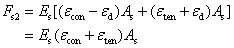(20)

where ed is strain difference between the edge and concentrated longitudinal reinforcement, r is the reinforcement ratio of the distributed longitudinal reinforcement, and As is the area of the concentrated reinforcement.

According to Fig. 7a, x can be calculated using Eq. 21. Letting econ or eten be equal to the yield strain ey, and solving the simultaneous Eqs. 17 to 21, the value of x can be obtained. Subsequently, the curvature limit of the yield point can be computed using Eq. 16.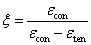(21)

3.3 Story curvature limit of the ultimate point (DS3)

The ultimate point adopted in this study is defined by the compressive concrete reaching the ultimate compressive strain ecu or the tensile reinforcement approaching the ultimate tensile strain esu. Given the ecu and esu, the story curvature limit of the ultimate point kultimate can be calculated using Eq. 22.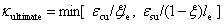(22)

As evident in Eq. 22, x is also crucial for the calculation of kultimate. The determination of x can follow the same procedures presented in Section 3.2, except that the stresses of the reinforcements are no longer linearly distributed. If the elasticĘCperfectly plastic stressĘCstrain relationship is adopted, the stress distribution of reinforcements is shown in Fig. 9. Hence, the internal normal force contributed by the reinforcements can be computed using Eq. 23.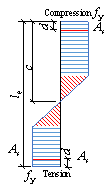Fig. 9.  Reinforcement stress distribution at the ultimate point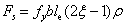(23)

Let econ be equal to ecu or eten be equal to esu, and solve the simultaneous Eqs. 17, 18, 21, and 23; then, the value of x can be obtained. Subsequently, the curvature limit of the ultimate point can be computed using Eq. 22.

4. Validation

The proposed method is validated using the refined FE models in Sections 4.1 and 4.2, and it is further validated by comparing it with the experimental results of six shear wall panels and a seven-story shear wall structure in Sections 4.3 and 4.4, respectively.

4.1 Comparison of the proposed method with the FE model of shear wall panels

x is a very important factor in the proposed method, the fiber beam model is adopted in this section as the benchmark model to validate the calculation of x. The fiber beam model can accurately simulate the flexural behavior of shear wall panels, and it has been extensively used in previous studies [33,34].

The dimension of the benchmark shear wall model has a height of 2 m, length of 1 m, and thickness of 0.1 m. The fiber beam model is implemented using the multi-purpose FE software MSC.MARC with the THUĘCfiber subroutine proposed by Lu et al. . The fiber element is divided into 36 segments along the length of the section. The stressĘCstrain relationship of Hognestad  is adopted for concrete fibers (Fig. 8) and the elasticĘCperfectly plastic stressĘCstrain relationship is used for steel fibers. Note that determining the material parameters is not the main topic of this study. Correspondingly, the concrete parameters fcp = 20 MPa, fcu = 17 MPa, ecy = 0.002, and ecu = 0.008 are adopted in all the cases. The influences of the axial load ratio, reinforcement ratio, and the strength of the longitudinal reinforcement are discussed herein. The parameters of all the cases are listed in Table 1. The result comparison is presented in Fig. 10, where í░Case1ĘC6-FEMí▒ indicates the results of the fiber beam model for Cases 1 to 6; while í░Case1ĘC6-Proposedí▒ represents the results of the proposed method for Cases 1 to 6.

Table 1. Model parameters

 Label Axial load ratio m Reinforcement ratio r Strength of the reinforcement fy Case 1 0.1ĘC0.5 0.5% 276 MPa Case 2 0.1ĘC0.5 0.5% 413 MPa Case 3 0.1ĘC0.5 0.5% 517 MPa Case 4 0.3 0.3%ĘC1.5% 276 MPa Case 5 0.3 0.3%ĘC1.5% 413 MPa Case 6 0.3 0.3%ĘC1.5% 517 MPa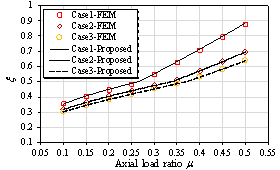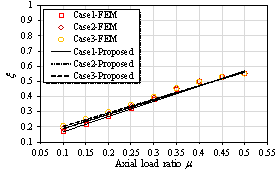(a) Cases 1ĘC3: Yield point (b) Cases 1ĘC3: Ultimate point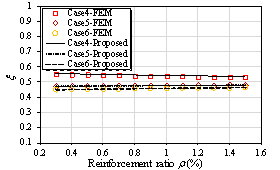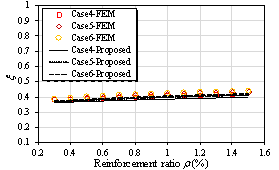(c) Cases 4ĘC6: Yield point (d) Cases 4ĘC6: Ultimate point

Fig. 10. Comparison of x with different shear wall parameters

As shown in Fig. 10, the results of the proposed method agree well with those of the fiber beam model, thereby validating the accuracy of the proposed method. The results of Figs. 10a and 10c indicate that the value of x at the yield point is mainly influenced by the axial load ratio and strength of the reinforcement. Moreover, the results of Figs. 10b and 10d show that the value of x at the ultimate point is also mainly controlled by the axial load ratio.

To validate the story curvature calculation and damage limit determination methods, a 20-story RC frameĘCshear wall building (Fig. 11) designed by Kuang and Huang  is simulated in this section. The axial load ratio of the bottom wall is set to 0.2. The material parameters fcp = 32 MPa, fcu = 27.2 MPa, ecy = 0.002, ecu = 0.008, and fy = 460 MPa are adopted. Other design parameters of the building are listed in Table 2. The MSC.MARC software with the THUĘCFiber beam model  are used to simulate the RC frame and shear wall components.Fig. 11. Schematic of the 20-story building

Table 2. Buildingí»s design parameters

 Structural element Section dimension (b í┴ h) (mm) Reinforcement ratio (%) Wall 300 í┴ 6000 1.0 Column 500 í┴ 500 0.6 Beam 250 í┴ 500 1.6

To validate the proposed story curvature calculation method, the nonlinear timeĘChistory analysis is performed using the El-Centro NS ground motion record  scaled from 100 gal to 800 gal. The story displacement results of the FE model are used as the input to calculate the mean story curvature by using the method proposed in Section 2. The mean story curvature results calculated by the FE model and the proposed method are compared in Fig. 12. Based on the comparison, the proposed method can accurately predict the story curvature.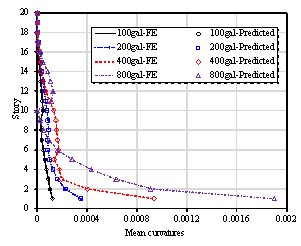Fig. 12.  Comparison of the mean story curvature of the FE model and predicted results

Fig. 13 demonstrates the curvature distribution of the 20-story building at the ultimate point of the pushover analysis. In Fig. 13, the piecewise linear distribution calculated using the proposed method is compared to the finite element results. The results indicate that damage concentration occurs at the bottom of the shear wall. Nevertheless, the piecewise linear distribution adopted in this study can reasonably simulate the curvature distribution in the shear wall.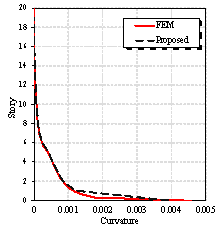Fig. 13.  Comparison of shear wall curvature distributions

To further validate the damage limits determination method, pushover analyses by using the force distribution of the first and second modal shapes are performed for the 20-story building. The crack, yield, and ultimate points of the FE model are presented as the square points highlighted in red in Fig. 14. Meanwhile, the maximum story curvatures of each story are calculated according to the method presented in Section 2, and the story curvature limits are also computed using the method in Section 3. The predicted crack, yield, and ultimate points are shown as the triangular points highlighted in green in Fig. 14. As shown in the figure, the proposed method can accurately estimate the damage states of the shear wall component of the building.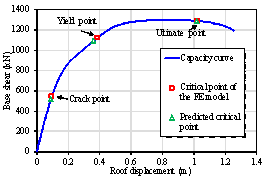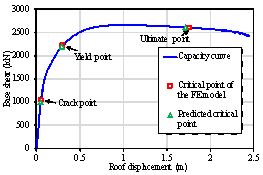(a) Modal shape 1 (b) Modal shape 2

Fig. 14. Damage assessment of using the FE model and the proposed method

The seismic damage assessment method of FEMA P58  is implemented to assess the damage state of the shear wall in the 20-story building. In Fig. 14a, the building is subjected to the lateral force of the first modal shape distribution. When attaining the ultimate point of the shear wall (concrete crushing), the effective height of the shear wall can be calculated as the ratio of the base moment to the base shear, which is 51.8 m, and the corresponding effective drift ratio is 0.0166. According to the FEMA P58 fragility database, the effective drift ratio limit of the DS3 damage state (core concrete damage) is 0.0186 for slender concrete shear walls, which is very close to the calculated result of 0.0166. This agreement indicates that the proposed method can reasonably estimate the damage at the bottom of the shear wall. For the second load case in Fig. 14b, the building is subjected to the lateral force of the second modal shape distribution. The calculated effective height at the ultimate point is 6.4 m and the effective drift ratio is 0.0024, which is significantly smaller than the effective drift ratio limit of DS3 (0.0186). According to the seismic damage assessment method of FEMA P58, the shear wall is not damaged. However, based on the proposed method, the ultimate point is attained, and the shear wall is severely damaged. This is because, under the lateral force of the second modal shape distribution, the bottom of the shear wall is not damaged, and damage occurs in the middle region of the shear wall. However, the FEMA P58 seismic damage assessment method using the effective drift ratio as the EDP can only consider the damage at the bottom of the shear wall. It should be noted that, for high-rise buildings, the higher-order vibration modes may contribute significantly to the damage of shear walls, and the damage in the middle region of the shear walls induced by higher-order vibration modes cannot be ignored. Compared to the seismic damage assessment of FEMA P58, the proposed method not only can assess the damage at the bottom of shear walls, but also reliably considers the higher-order vibration-induced damage in the middle region of shear walls.

Subsequently, the timeĘChistory analyses by using the El-Centro NS ground motion record are performed for the 20-story building. The damage states calculated by using the FE model and the proposed method are compared in Fig. 15. The comparison shows good agreement, thereby validating the proposed method. Notably, in the case of ground motion record scaled to 800 gal, the proposed method can not only predict the DS2 damage at the bottom but also identify the DS2 damage at the middle region (8ĘC11 story) of the shear wall caused by higher modal vibrations.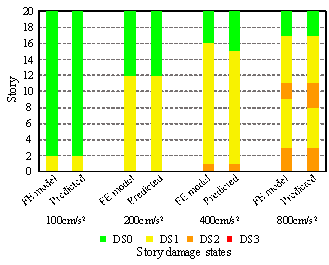Fig. 15. Damage state comparison of the FE model and proposed method under the excitation of El-Centro NS ground motion record

Finally, timeĘChistory analyses of the building subjected to 22 far-field ground motion records are performed as recommended by FEMA . The PGAs of all the 22 ground motion records are scaled to 400 gal. The comparative results are presented in Fig. 16. The comparison also shows good agreement, hence further validating the accuracy and reliability of the proposed method.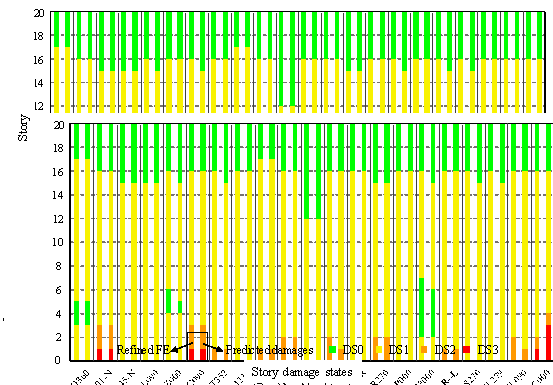Fig. 16.  Damage state comparison of the FE model and proposed method under the excitation of 22 far-field ground motion records (The x-axis is the name of different ground motion records)

4.3 Comparison with the experimental results of shear wall panels

To validate the proposed damage limit determination method, the experimental results of six half-scale shear wall specimens are used for the comparison. All the specimens have a length of 2 m and height of 4.56 m . The reinforcement layouts of specimens WSH1ĘCWSH6 are presented in Fig. 17 and the detailed specimen parameters are summarized in Table 3.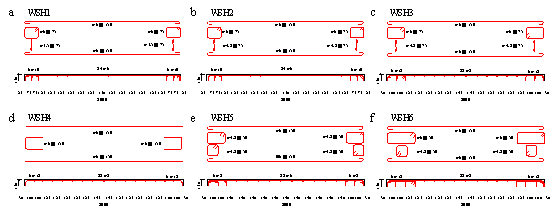Fig. 17.  Reinforcement layouts in the plastic zone of the test units (unit: mm) 

Table 3. Parameters of all the specimens

 Test unit fy (MPa) fcp (MPa) m rbound (%) rweb (%) Agt (%) WSH1 547.3 45.0 0.051 1.32 0.30 4.64 WSH2 583.1 40.5 0.057 1.32 0.30 7.71 WSH3 601.0 39.2 0.058 1.54 0.54 7.69 WSH4 576.0 40.9 0.057 1.54 0.54 7.29 WSH5 576.0 38.3 0.128 0.67 0.27 7.29 WSH6 576.0 45.6 0.108 1.54 0.54 7.29

where fy is the yield strength of reinforcements; fcp is the cylinder strength of concrete; m is the axial load ratio; rbound and rweb are the reinforcement ratios of the boundary element and the web, respectively; and Agt is the reinforcement elongation at maximum force.

Strain gauges are installed in the reinforcement. The strain results are used to define the yield point of each specimen. According to the experimental results, the ultimate points of specimens WSH1ĘCWSH3 and WSH5ĘCWSH6 are controlled by 0.6Agt . The specimen WSH4 does not have sufficient stirrup to confine the compressive concrete. Consequently, its ultimate point is controlled by a crushing strain of 0.004.

Based on the reinforcement ratios of the web and boundary elements, the axial load ratio, and the material strengths presented in Table 3, the curvature limit of the yield point of each specimen can be calculated using Eqs. 16 to 21. The curvature limit of the ultimate point of each specimen can be computed according to Eqs. 17, 18, 21, 22, and 23. The comparison of the curvature limits of the yield and ultimate points are shown in Fig. 18. The elicited results also show good agreement.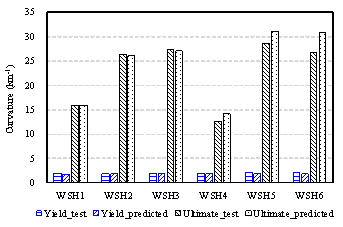Fig. 18. Results of the yielding and ultimate point curvatures elicited from the experiments and the proposed method

4.4 Comparison with the experimental result of a shear wall structure

In this section, the shaking table test of a full-size, seven-story shear wall building, performed by the University of California, San Diego (UCSD), the Portland Cement Association (PCA) of Skokie (IL), and the NEES Consortium Inc. (NEESinc)  are investigated to validate the proposed model. The experimental details are illustrated in Fig. 19. The web shear wall is the main object of the shaking table test. The flange wall and PT segmental pier are used to ensure the out-of-plane stability of the web wall.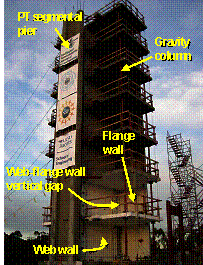Fig. 19. Shaking table test of the full-size, seven-story shear wall building 

The building has been subjected to four different levels of ground motions (EQ1ĘC4). The damages at the bottom of the web wall can be presented by the ductility factor (ratio of maximum strain to the yielding strain) of the reinforcement in the boundary element . The ductility factors at different intensity levels are presented in Fig. 20.

To apply the proposed method, the story curvature at the bottom of the shear wall was calculated using the story drift results of the experiments. Subsequently, according to Eqs. 16ĘC21 presented in section 3.2, the curvature limit of the yield point was calculated using the shear wall key design parameters as shown in Table 4 [40,41]. Finally, the ductility factors (ratio of the maximum story curvature to the yield story curvature) at the bottom of the shear wall were computed for the four different intensity levels, as shown in Fig. 20. As presented in the figure, the proposed method can reasonably estimate the extent of the damage of the seven-story shear wall structures, thereby further validating the reliability of the proposed method.

Table 4. Key design parameters in the first story of the web wall

 fy (MPa) fcp (MPa) m rbound (%) rweb (%) 450 37.4 0.044 2.21 0.308

where fy is the yield strength of reinforcements; fcp is the cylinder strength of concrete; m is the axial load ratio; rbound and rweb are the reinforcement ratios of the boundary element and the web, respectively.Fig. 20. Ductility factor comparison at the bottom of the shear wall